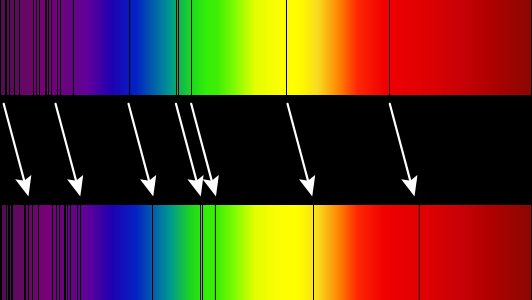# 7.3: Redshift

$$\newcommand{\vecs}{\overset { \rightharpoonup} {\mathbf{#1}} }$$ $$\newcommand{\vecd}{\overset{-\!-\!\rightharpoonup}{\vphantom{a}\smash {#1}}}$$$$\newcommand{\id}{\mathrm{id}}$$ $$\newcommand{\Span}{\mathrm{span}}$$ $$\newcommand{\kernel}{\mathrm{null}\,}$$ $$\newcommand{\range}{\mathrm{range}\,}$$ $$\newcommand{\RealPart}{\mathrm{Re}}$$ $$\newcommand{\ImaginaryPart}{\mathrm{Im}}$$ $$\newcommand{\Argument}{\mathrm{Arg}}$$ $$\newcommand{\norm}{\| #1 \|}$$ $$\newcommand{\inner}{\langle #1, #2 \rangle}$$ $$\newcommand{\Span}{\mathrm{span}}$$ $$\newcommand{\id}{\mathrm{id}}$$ $$\newcommand{\Span}{\mathrm{span}}$$ $$\newcommand{\kernel}{\mathrm{null}\,}$$ $$\newcommand{\range}{\mathrm{range}\,}$$ $$\newcommand{\RealPart}{\mathrm{Re}}$$ $$\newcommand{\ImaginaryPart}{\mathrm{Im}}$$ $$\newcommand{\Argument}{\mathrm{Arg}}$$ $$\newcommand{\norm}{\| #1 \|}$$ $$\newcommand{\inner}{\langle #1, #2 \rangle}$$ $$\newcommand{\Span}{\mathrm{span}}$$$$\newcommand{\AA}{\unicode[.8,0]{x212B}}$$

In the previous section we saw that the proper distance between two objects can increase or decrease with time depending on the function $$a(t)$$, even if the objects are "stationary". What if those two "objects" are the crests of a light wave? As the light wave travels, then distance between those two crests can change. This causes the wavelength, and therefore the frequency, of the wave to change. We refer to this as cosmological redshift.

## Definition: Cosmological Redshift

Cosmological redshift is the increase in wavelength that light undergoes as a result of traveling through an expanding universe.

Note

Redshift has two other causes in addition to the expansion of the universe. The first is due to peculiar velocity, which is the local motion of a star. If we were to look at a galaxy edge-on, for example, stars on one side would be moving toward us while stars on the other side would be moving away from us. Light from the stars moving toward us would be blueshifted while light from stars moving away from us would be redshifted. The other source of redshift is called gravitational redshift, which results when light loses energy by climbing out a gravitational potential energy well (as we saw with the infinite redshift surface of a black hole).

We will see later that $$a(t)$$ increases with time, which increases the wavelength and "reddens" it, as shown in Figure $$\PageIndex{1}$$.Figure $$\PageIndex{1}$$: Absorption lines of the sun as compared with absorption lines from a distant supercluster of galaxies. (Image credit: Wikipedia)

Redshift $$z$$ is the fraction by which the wavelength $$\lambda$$ increases. We write this as

$\begin{equation}\lambda_0=(1+z)\lambda_\text{emit}\label{eq:WavelengthRedshift}\end{equation}$

where $$\lambda_\text{emit}$$ is the emitted wavelength, $$\lambda_0$$ is the received wavelength (once again using the subscript "0" to represent "today"), and $$z$$ is the redshift. The factor $$1+z$$ is often called the stretch factor. Due to the fact that proper distances scale with the scale factor, it can be shown that

$1+z=\frac{\lambda_0}{\lambda_\text{emit}}=\frac{a(t_0)}{a(t_\text{emit})}.\label{eq:StretchFactor}$

Note that, according to Equation \ref{eq:WavelengthRedshift}, in order to determine redshift based on the measured wavelength of the received light, you must know the wavelength of the light when it was emitted. For monochromatic light, that is very difficult to determine. Fortunately, stars emit light with a predictable pattern of discrete wavelengths. Light from stars that are far away has the same pattern but shifted toward the red end of the spectrum, as shown in Figure $$\PageIndex{1}$$. So redshift is actually very easy for astronomers to determine.

## Note

If you spend any amount of time with astronomers, you will find that they often use redshift as a proxy for time or distance. That is, they will use redshift to refer to how old or how far away something is (or was). Redshift is related to how old and how far away something is, but distances and times require knowing $$a(t)$$, while redshift does not.

## Box $$\PageIndex{1}$$

Some of the most distant observed objects emitted light so long ago that the light is redshifted by $$z=6$$.

1. If the light was emitted in the visible range of the spectrum, in what range of the spectrum do we receive it?
2. What was the scale factor at the time of emission?
3. At what time was the light emitted? Use $$a(t)=\frac{1}{t_0}t$$.
4. How far away was the light source when it emitted the light?
5. How far away is the light source now?

7.3: Redshift is shared under a not declared license and was authored, remixed, and/or curated by LibreTexts.